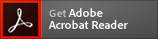Bacitracin zinc salt CAS: 1405-89-6, MF: C66H101N17O16SZn, MW: 1486.07

# Bacitracin zinc salt (CAS 1405-89-6)

CAS Number: 1405-89-6
Molecular Weight: 1486.07
Molecular Formula: C66H101N17O16SZn
* Refer to Certificate of Analysis for lot specific data (including water content).Appearance :
Powder
Physical State :
Solid
Solubility :
Soluble in 1 M HCl: 50 mg/mL
Storage :
Store at 4° C
Melting Point :
~250° C
For Research Use Only. Not Intended for Diagnostic or Therapeutic Use.
PubChem CID :
16219032
SMILES :
CC[C@H](C)[C@H]1C(=O)N[C@@H](C(=O)N[C@H](C(=O)N[C@@H](C(=O)N[C@H](C(=O)NCCCC[C@@H](C(=O)N[C@@H](C(=O)N1)CCCN)NC(=O)[C@H]([C@@H](C)CC)NC(=O)[C@@H](CCC(=O)[O-])NC(=O)[C@H](CC(C)C)NC(=O)C2CSC(=N2)C(C(C)CC)N)CC(=O)N)CC(=O)[O-])CC3=CN=CN3)CC4=CC=CC=C4.[Zn+2]

#### Certificate of Analysis